# CSCI 3328 Object Oriented Programming in C Chapter

• Slides: 15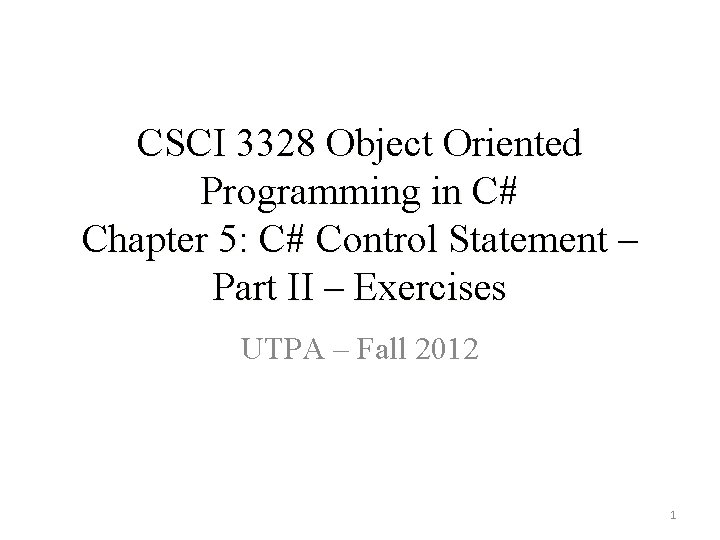CSCI 3328 Object Oriented Programming in C# Chapter 5: C# Control Statement – Part II – Exercises UTPA – Fall 2012 1Objectives • In this chapter, you will do exercises about: – more control structures • Repetition statements – for, do … while • Selection – Switch – break and continue statements – logical operators in C# 2Multiple Choices • Typically, ______ statements are used for counter-controlled repetition. – A. for B. while C. repetition D. until • Typically, ______ statements are used for sentinel-controlled repetition. – A. for B. while C. repetition D. until • The do … while statement tests the loop-continuation condition ____ executing the loop’s body; therefore, the body always executes at least once. – A. before B. after C. at the same time D. None of the above • The _____statement, when executed in a repetition statement, skips the remaining statements in the loop body and proceeds with the next iteration of the loop. – A. break B. exit C. continue D. return 3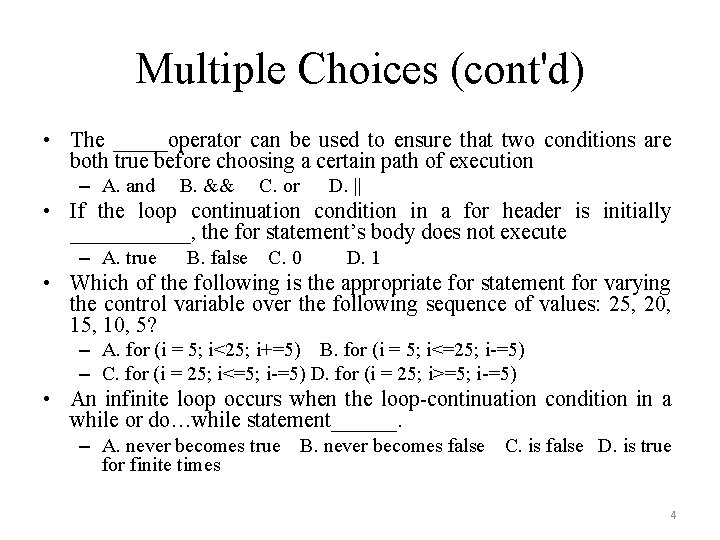Multiple Choices (cont'd) • The _____operator can be used to ensure that two conditions are both true before choosing a certain path of execution – A. and B. && C. or D. || • If the loop continuation condition in a for header is initially ______, the for statement’s body does not execute – A. true B. false C. 0 D. 1 • Which of the following is the appropriate for statement for varying the control variable over the following sequence of values: 25, 20, 15, 10, 5? – A. for (i = 5; i<25; i+=5) B. for (i = 5; i<=25; i-=5) – C. for (i = 25; i<=5; i-=5) D. for (i = 25; i>=5; i-=5) • An infinite loop occurs when the loop-continuation condition in a while or do…while statement______. – A. never becomes true B. never becomes false C. is false D. is true for finite times 4True/False Statements • The default label is required in the switch selection statement. • The break statement is required in every case of a switch statement • The expression ((x>y)&&(a<b)) is true if either (x>y) is true or (a<b) is true. • An expression containing the || operator is true if either or both of its operands are true. • The integer after the comma (, ) in a format item (e. g. , {0, 4}) indicates the field width of the displayed string. • To test for a range of values in a switch statement, use a hyphen (-) between the start and end values of the range in a case label. 5Recall: Example of Multiple Selection Statement public void Calculate. Grade(int grade) { switch (grade/10) { case 9: case 10: Console. Write. Line("Grade: A"); break; case 8: Console. Write. Line("Grade: B"); break; case 7: Console. Write. Line("Grade: C"); break; case 6: Console. Write. Line("Grade: D"); return; default: Console. Write. Line("Grade: F"); break; } } // 90 -99 // 100 // 80 -89 // 70 -79 // 60 -69 // < 60 6True/False Statements (cont'd) • Listing cases consecutively with no statements between them enables the cases to perform the same set of statements. • Boolean logical operator (|) performs short-circuit evaluation. • The && operator has a higher precedence than the || operator. • The following statement alters the control variable from 0 to 50 in increment of 5? – for (int i=0; i<49; i+=5) 7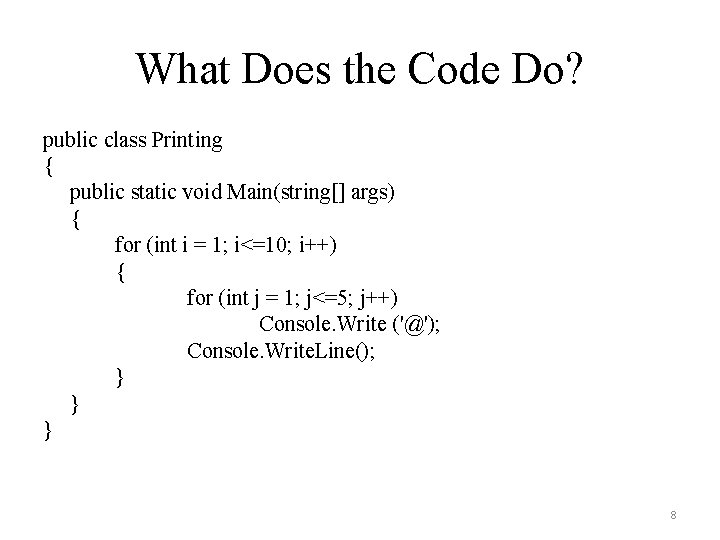What Does the Code Do? public class Printing { public static void Main(string[] args) { for (int i = 1; i<=10; i++) { for (int j = 1; j<=5; j++) Console. Write ('@'); Console. Write. Line(); } } } 8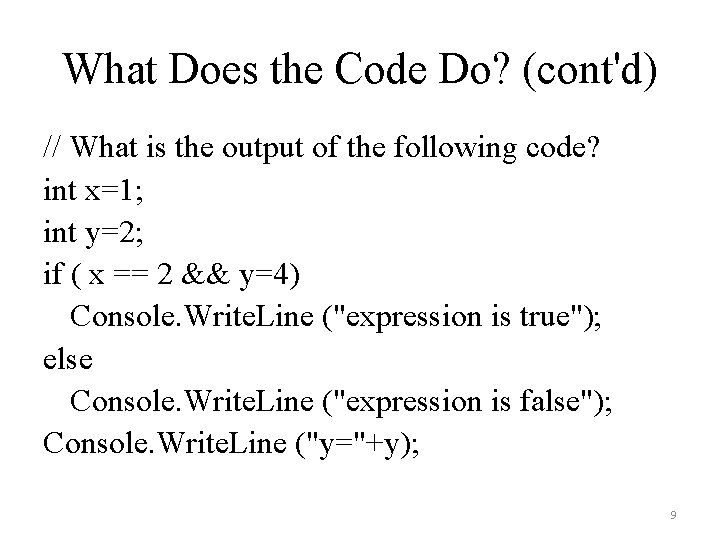What Does the Code Do? (cont'd) // What is the output of the following code? int x=1; int y=2; if ( x == 2 && y=4) Console. Write. Line ("expression is true"); else Console. Write. Line ("expression is false"); Console. Write. Line ("y="+y); 9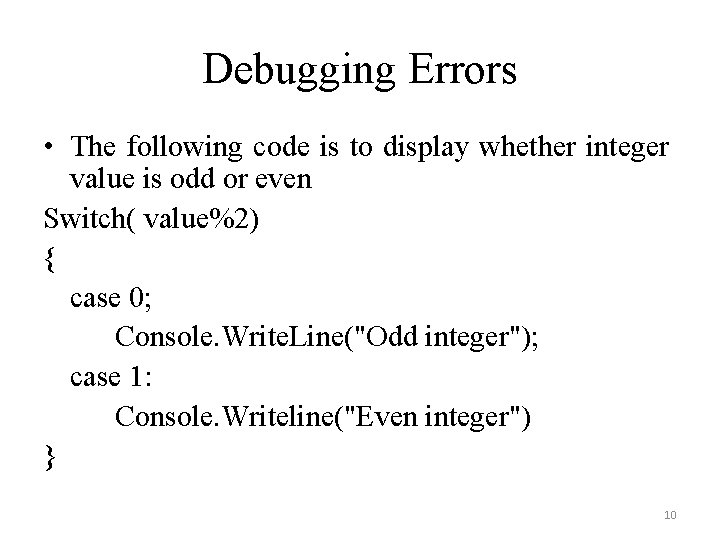Debugging Errors • The following code is to display whether integer value is odd or even Switch( value%2) { case 0; Console. Write. Line("Odd integer"); case 1: Console. Writeline("Even integer") } 10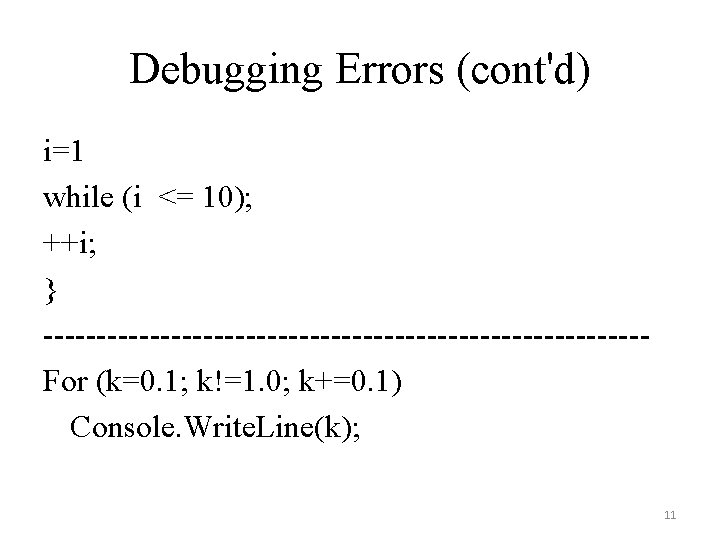Debugging Errors (cont'd) i=1 while (i <= 10); ++i; } ----------------------------For (k=0. 1; k!=1. 0; k+=0. 1) Console. Write. Line(k); 11Write a Program • Use nested for statement to write a program to display the triangle of asterisks in output. Text. Box. Use the following statements: – output. Text. Box. Append. Text("*"): displays asterisks one at a time – output. Text. Box. Append. Text(Environment. New. Line) – output. Text. Box. Append. Text(" "): inserts a space * ** ****** ********** ********* ***** *** ********* ***** *** ** **** ******** ********** 12Write a Program (cont'd) • Use nested for statement to write a program to display a rectangle in output. Text. Box. Use the following statements: – output. Text. Box. Append. Text("+") – output. Text. Box. Append. Text(Environment. New. Line) – output. Text. Box. Append. Text(" "): inserts a space +-----+ | | | +-----+ 13Exercises After the Class • Chapter 6 in your textbook – Self-Review Exercises – Quick Quiz – Exercises 1415